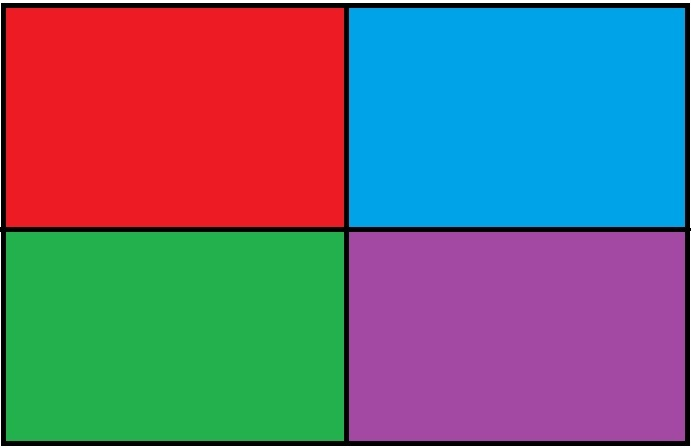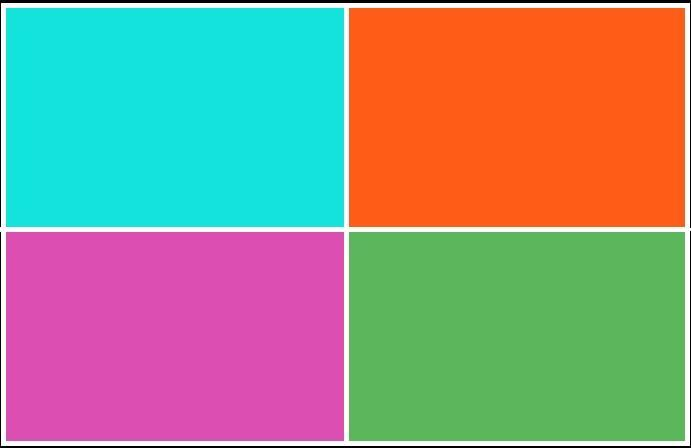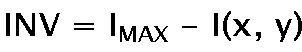Related Articles

# Python – Color Inversion using Pillow

• Last Updated : 22 Apr, 2020

Color Inversion (Image Negative) is the method of inverting pixel values of an image. Image inversion does not depend on the color mode of the image, i.e. inversion works on channel level. When inversion is used on a multi color image (RGB, CMYK etc) then each channel is treated separately, and the final result if formed by callibrating the results of all the channels.

We would be using pillow (`PIL`) library for obtaining negative of an image. To install the library, execute the following command in the command-line:-

```pip install pillow
```

Note: Several Linux distributions tend to have Python and PIL preinstalled into them.

In this article, 2 methods have been described for inverting color space of an image. The first one is an inbuilt method using `ImageChops.invert()` function. In the second one we would be inverting the image by elementwise substraction of pixel values.

Sample Image –Method #1:

Using inbuilt method `ImageChops.invert()` for Negating color.

 `# Importing imagechops for using the invert() method``from` `PIL ``import` `Image, ImageChops`` ` `# Opening the test image, and saving it's object``img ``=` `Image.``open``(``'test.jpg'``)`` ` `# Passing the image object to invert()  ``inv_img ``=` `ImageChops.invert(img)`` ` `# Displaying the output image``inv_img.show()`

Output:Explanation:
Firstly we import the `ImageChops` module for using `invert()` method. Then we open the test image (`test.jpg`), and save it’s image object. Now we passed that image object to `ImageDraw.invert()` which returns the inverted image. At last, we displayed the color inverted image.

Things to keep in mind while using `ImageChops.invert()`:

• The input Image should not contain a Alpha channel

• The input image should not be of P (Paletted) color mode.

Method #2:

The method used for getting the inverse of an image is subtraction of the maximum value/intensity of a pixel by the value of current pixel. The resultant value is guided by the formula –Where `INV` is the resultant inverted pixel, `I^MAX` is the maximum intensity level in a given color mode and `I(x, y)` is the intensity (pixel value) of image/color channel at a particular pair of coordinates.

 `from` `PIL ``import` `Image`` ` `# numpy for performing batch processing and elementwise``# matrix operations efficiently``import` `numpy as np`` ` ` ` `# Opening an image, and saving open image object``img ``=` `Image.``open``(r``"sample.jpg"``)`` ` `# Creating an numpy array out of the image object``img_arry ``=` `np.array(img)`` ` `# Maximum intensity value of the color mode``I_max ``=` `255`` ` `# Substracting 255 (max value possible in a given image ``# channel) from each pixel values and storing the result``img_arry ``=` `I_max ``-` `img_arry`` ` `# Creating an image object from the resultant numpy array``inverted_img ``=` `Image.fromarray(img_arry)`` ` `# Saving the image under the name Image_negative.jpg``inverted_img.save(r``"Image_negative.jpg"``)`

Output:Explanation:

Firstly we import numpy into our code, as numpy allows fast elementwise operations on matrices and offers several arithmetic operations on arrays. Then we open the test image using `Image.open()`, and store the returned image object in the variable `img`. Then we create an array (`img_arry`) from the pixel values obtained from the opened image object (`img`). This is done so as to allow elementwise substraction operation offered by the numpy library. Now we substract 255 from each channel/pixel value, this results in all the pixel values being inverted. Now, we use this resultant matrix to create an new image (`inverted_img`). Finally we saved the image, under the name of `Image_negative.jpg`.

### Some things to keep in mind –

• It should be ensured that the input image does not contain an alpha channel. This is because when the line `img_arry = 255 - img_arry` will execute for an image containing alpha channel, it would invert the alpha channel values as well. This would lead to inconsistency in the output image, as we may end up with completly transparent images (which is not a part of color inversion). One way to allow processing `RGBA` images is firstly converting them to `RGB` color mode using `Image.convert('RGB')`. Alternatively, we could extract the alpha channel using `Image.getdata(band=3)` and then later combine it to the final image to get back the original `RGBA` image.

This input image `sample.jpg` is chosen intentionally of the format `.jpg` as a JPG/JPEG image format doesn’t support transparency or alpha channels.

• Input image should not be of `P` (paletted) mode. As a paletted image does not contain pixel values at coordinates, but rather index to pixel values belonging to a color map (of varying sizes). Therefore, image inversion would lead to inconsistent results.

• The value `I_max = 255` is assigned assuming that the maximum intensity achievable in the specific image mode is 255. The value isn’t hardcoded. The value depends on the color mode, and therefore could be less then 255 (ex. `1` in bilevel image) or greater than 255 (ex. `32536` in 16 Bit Unsigned Greyscale mode).

Attention geek! Strengthen your foundations with the Python Programming Foundation Course and learn the basics.

To begin with, your interview preparations Enhance your Data Structures concepts with the Python DS Course. And to begin with your Machine Learning Journey, join the Machine Learning – Basic Level Course

My Personal Notes arrow_drop_up